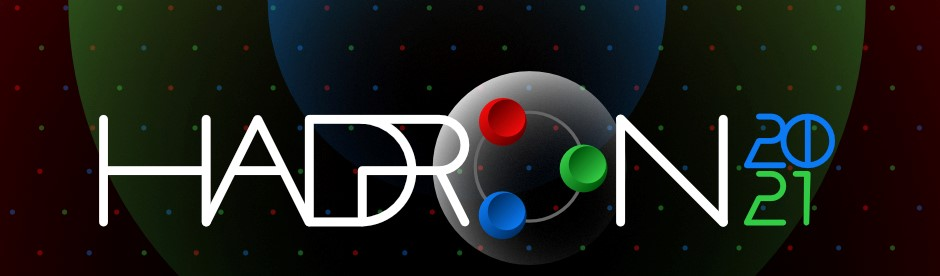#19th International Conference on Hadron Spectroscopy and Structure in memoriam Simon Eidelman

26-31 July 2021
Mexico City
Mexico/General timezone
HADRON 2021 is over. Thanks for making it a success!
Home > Timetable > Session details > Contribution details

Mexico City -

# The effect of hidden-charm strange pentaquarks $P_{cs}$ on the $K^- \,p \to J/\psi \,\Lambda$ reaction

## Speakers

• Mr. Samson CLYMTON

## Abstract

We present a recent investigation on the production of $P_{cs}(4459)$ in the exclusive $K^-p\to J/\psi\Lambda$ reaction using both the effective Lagrangian and Regge approaches. We assume six different spin and parity assignments, i.e., $1/2^\pm$, $3/2^\pm$ and $5/2^\pm$ to the newly found $P_{cs}$. The total and differential cross sections for the $K^-p\to J/\psi\Lambda$ reaction are calculated. We examine the dependence of the results on different assignments of $J^P$ for $P_{cs}$.